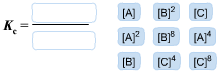# Problem: Consider the equilibrium reaction 2A + B ⇌ 4CAfter multiplying the reaction by a factor of 2, what is the new equilibrium equation? Drag the following labels into the numerator and denominator as needed to create the equilibrium-constant expression, Kc, for the new equilibrium reaction, If the initial reaction contains 1.31 M A, 1.21 M B, and 2.51 M C, calculate K0 for the new equilibrium reaction.Kc =

###### FREE Expert Solution

We are asked what is the new equilibrium equation after multiplying the reaction by a factor of 2.

2A + B ⇌ 4C

multiply by 2:

(2A + B ⇌ 4C) x 2

4A + 2B ⇌ 8C

Get the Kc expression:

The new Kc expression is:

97% (438 ratings)###### Problem Details

Consider the equilibrium reaction

2A + B ⇌ 4C

After multiplying the reaction by a factor of 2, what is the new equilibrium equation?

Drag the following labels into the numerator and denominator as needed to create the equilibrium-constant expression, Kc, for the new equilibrium reaction,If the initial reaction contains 1.31 M A, 1.21 M B, and 2.51 M C, calculate K0 for the new equilibrium reaction.

Kc